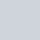Latest MCQs
Home > MCQs > Math > The ages of C and D are in 6:11 and E is 6 years younger than D. If the sum of ages is 134 then what is the age of D?

# The ages of C and D are in 6:11 and E is 6 years younger than D. If the sum of ages is 134 then what is the age of D?

A.65
B.70
C.35
D.55